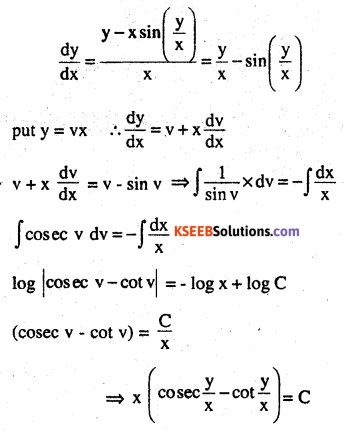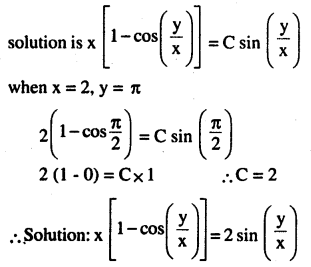# 2nd PUC Maths Question Bank Chapter 9 Differential Equations Miscellaneous Exercise

Students can Download Maths Chapter 9 Differential Equations Miscellaneous Exercise Questions and Answers, Notes Pdf, 2nd PUC Maths Question Bank with Answers helps you to revise the complete Karnataka State Board Syllabus and score more marks in your examinations.

## Karnataka 2nd PUC Maths Question Bank Chapter 9 Differential Equations Miscellaneous Exercise

Question 1.
For each of the differential equations given below, indicate its order and degree
(if defined).

(i)
$$\cfrac{d^{2} y}{d x^{2}}+5 x\left(\cfrac{d y}{d x}\right)^{2}-6 y=\log x$$
Order -2, degree – 1

(ii)
$$\left(\cfrac{d y}{d x}\right)^{3}-4\left(\cfrac{d y}{d x}\right)^{2}+7 y=\sin x$$
Order is 1 degree is 3.

(iii)
$$\cfrac{d^{4} y}{d x^{4}}-\sin \left(\cfrac{d^{3} y}{d x^{3}}\right)=0$$
Order is 4. As the equation is not a polynomial in $$\frac{d y}{d x}$$, degree is not defined.Question 2.
For each of the exercises given below verify that the given function (implicit or explicit) is a solution of the corresponding differential equation.

(i) y = a ex + b e-x + x2
$$x \cfrac{d^{2} y}{d x^{2}}+2 \cfrac{d y}{d x}-x y+x^{2}-2=0$$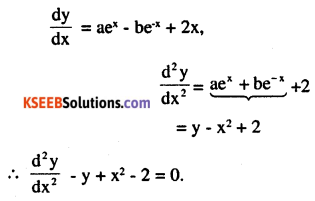(ii) y = ex (a cos x + b sin x)
$$\cfrac{d^{2} y}{d x^{2}}-2 \cfrac{d y}{d x}+2 y=0$$
$$\frac{d y}{d x}$$ = ex (-a sin x + b cos x) + (+a cos x + b sin x) ex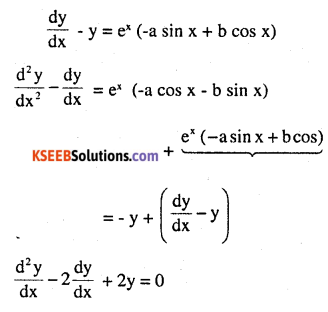(iii) $$y=x \sin 3 x \quad: \cfrac{d^{2} y}{d x^{2}}+9 y-6 \cos 3 x=0$$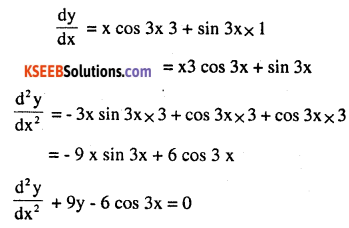(iv) $$2 x=2 y^{2} \times \cfrac{1}{y} \times \cfrac{d y}{d x}+\log y \times 4 y \times \cfrac{d y}{d x}$$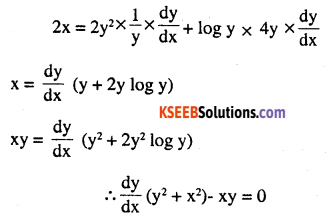Question 3.
Form the differential equation representing the family of curves given by
(x – a)2 + 2y2 = a2, where a is an arbitrary constant.
(x – a)2 + 2y2 = a2
2(x -a ) +2(2y) x $$\frac{d y}{d x}$$ = 0
(x – a) + 2yx y’ = 0, 2y y’ = – (x – a) = a – x ………….(1)
4y2 (y’ )2 + 2 y2 = 4y2 (y’ )2 + 4 xy y’ + x2
$$4 x y \frac{d y}{d x}+x^{2}-2 y^{2}=0$$

Question 4.
Prove that x2 – y2 = c (x2 + y2) is the general solution of differential equation (x3 – 3x y2) dx = (y3 – 3x2y) dy, where c is a parameter.
x2 – y2 = c (x2 + y2)2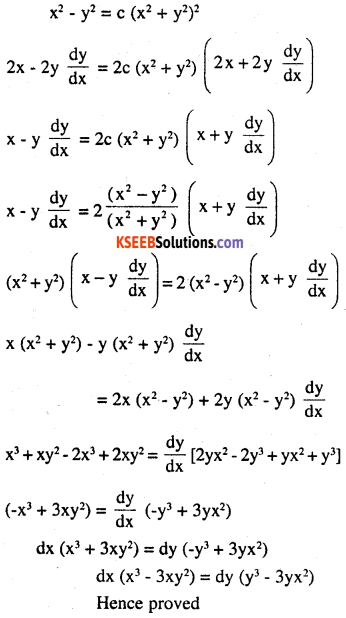Question 5.
Form the differential equation of the family of circles in the first quadrant which touch the coordinate axes.
(x – h)2 + (y – h)2 = h2 dy
2(x – h) +2(y – h) $$\frac{d y}{d x}=0$$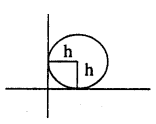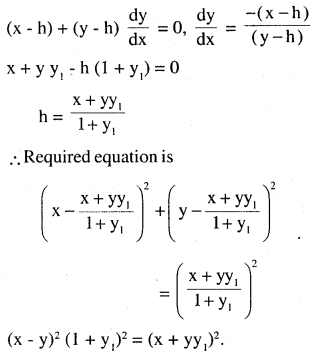Question 6.
Find the general solution of the differential equation
$$\cfrac{d y}{d x}+\sqrt{\cfrac{1-y^{2}}{1-x^{2}}}=0$$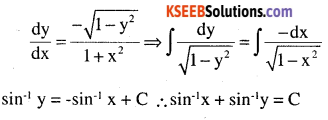Question 7.
Show that the general solution of the different equation
$$\frac{d y}{d x}+\frac{y^{2}+y+1}{x^{2}+x+1}=0$$ is given by
(x +y+1) = A (1- x – y -2xy)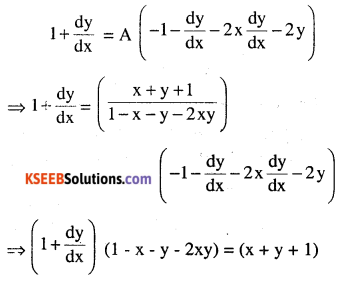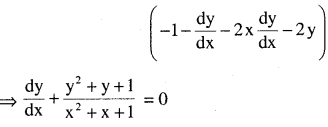Question 8.
Find the equation of the curve passing through the point $$\left(0, \frac{\pi}{4}\right)$$ whose differencial  equation is sin x y dy = 0
Sin x cos y dx = – cos x sin y dy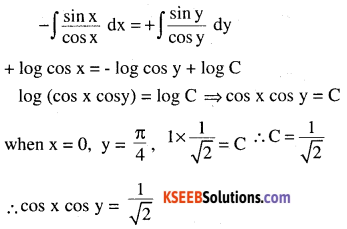Question 9.
Find the particular solution of the differential equation (1 + e2x) dy + (1 + y2) ex dx = 0, given that y = 1 when x = 0.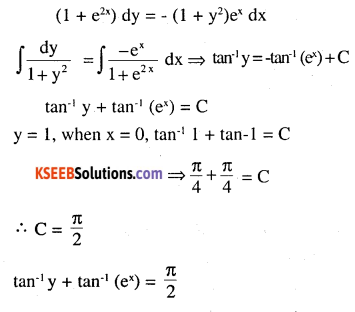Question 10.
Solve the differential equation
$$\mathbf{y}^{\mathbf{e}^{\frac{x}{y}}} \mathbf{d x}=\left(\mathbf{x e}^{\frac{\mathbf{x}}{y}}+\mathbf{y}^{2}\right) \mathbf{d y}(\mathbf{y} \neq \mathbf{0})$$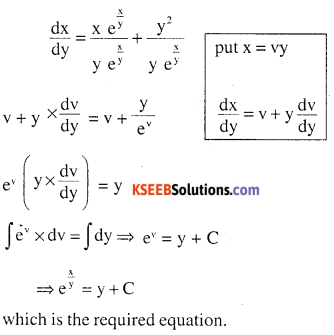Question 11.
Find a particular solution of the differential equation (x – y) (dx + dy) = dx – dy, given that y = -1, when x = 0. (Hint: put x – y = t)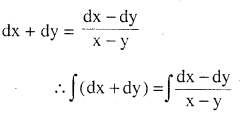x + y = log (x – y) + C
when x = 0, y = -1
0 – 1 = log(0 + 1) + C
⇒ C = -1 solution is x + y = log (x – y) – 1.Question 12.
Solve the differential equation
$$\left[\cfrac{\mathrm{e}^{-2 \sqrt{x}}}{\sqrt{x}}-\cfrac{y}{\sqrt{x}}\right] \cfrac{d x}{d y}=1(x \neq 0)$$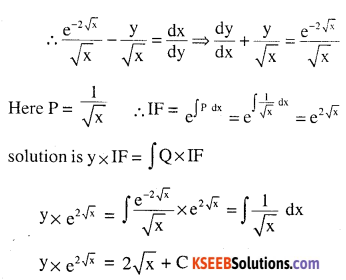Question 13.
Find a particular solution of the differential dy equation $$\frac{d y}{d x}+y \cot x=4 x \csc x(x \neq 0)$$ given that y = 0 when $$x=\frac{\pi}{2}$$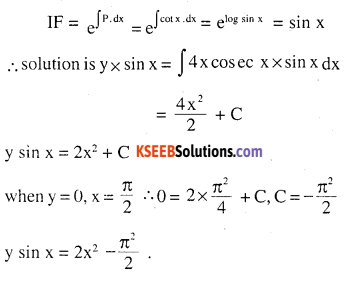Question 14.
Find a particular solution of the differential dy equation (x+1) \frac{d y}{d x}=2 e^{-y}-1, given that y = 0 when x = 0.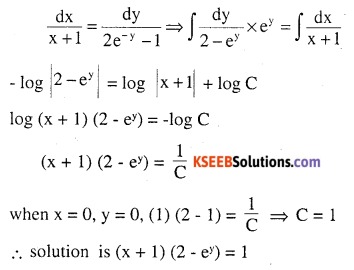Question 15.
The population of a village increases continuously at the rate proportional to the number of its inhabitants present at any time. If the population of the village was 20,000 in 1999 and 25000 in the year 2004, what will be the population of the village in 2009?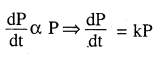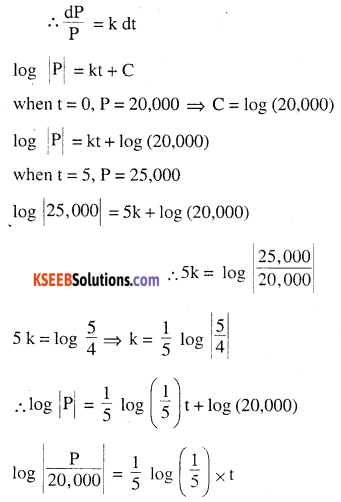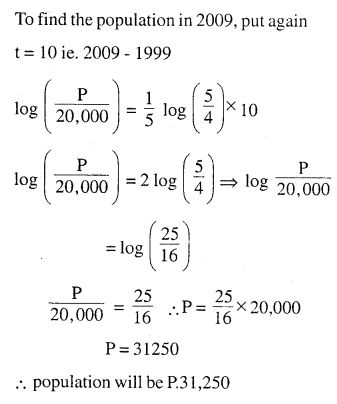Question 16.
The general solution of the differential equation $$\frac{y d x-x d y}{y}=0$$ is
(A) xy = C
(B) x = Cy2
(C) y = Cx
(D) y = Cx2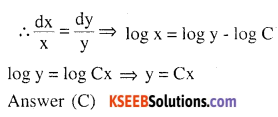Question 17.
The general solution of a differential equation of the type $$\frac{d x}{d y}+P_{1} x=Q_{1} \text { is }$$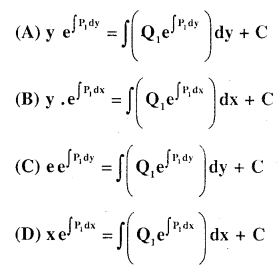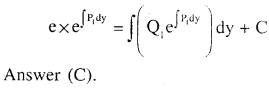Question 18.
The general solution of the differential equation ex dy + (y ex + 2x) dx = 0 is
(A) x ey+ x2 = C
(B) x ey + y2 = C
(C) y ex + x2 = C
(D) y ey + x2 = C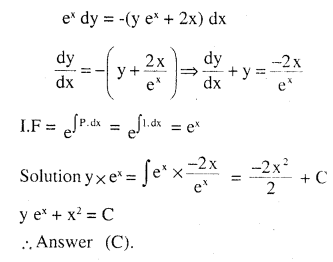### 2nd PUC Maths Differential Equations Miscellaneous Exercise Additional Questions and Answers

Question 1.
Solve the differential equations
$$\sqrt{1+x^{2}+y^{2}+x^{2} y^{2}}+x y \cfrac{d y}{d x}=0$$ (CBSE 2010)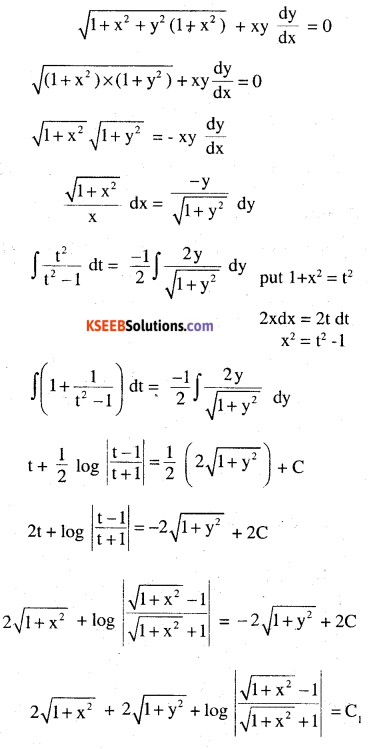Question 2.
solve
$$\left(x^{2}-1\right) \cfrac{d y}{d x}+2 x y=\cfrac{1}{x^{2}-1}$$(CBSE 2010)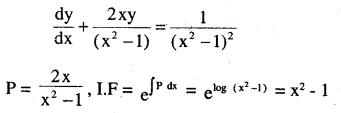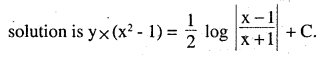Question 3.
Show that $$(x-y) \frac{d y}{d x}=x+2 y$$ is homogenous and solve it (CBSE 2010)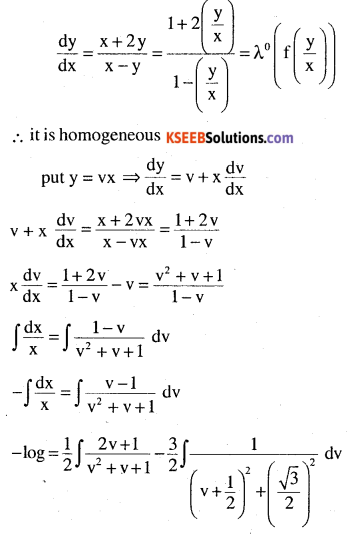Question 4.
Solve
$$y d x+x \log \left(\cfrac{y}{x}\right) d y-2 x d y=0$$ (CBSE 2010)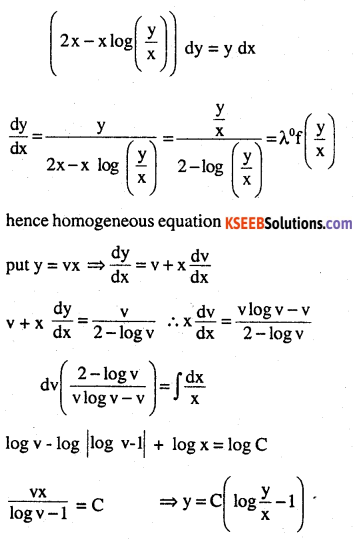Question 5.
solve
$$\left(x^{3}+x^{2}+x+1\right)\left(\cfrac{d y}{d x}\right)=2 x^{2}+x$$ (CBSE 2010)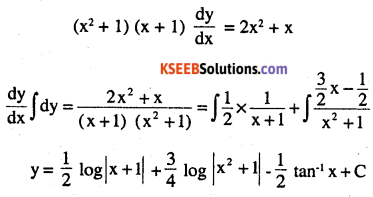Question 6.
Solve
$$\left(x^{2}+1\right) \cfrac{d y}{d x}+2 x y=\sqrt{x^{2}+4}$$(CBSE 2010)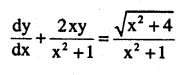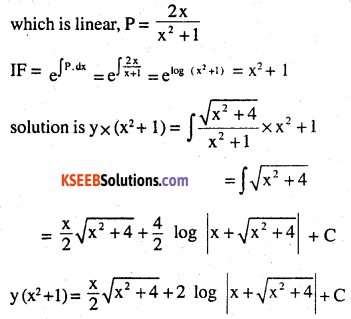Question 7.
Solve $$x d y-y d x=\sqrt{x^{2}+y^{2}} d x$$(CBSE 2011)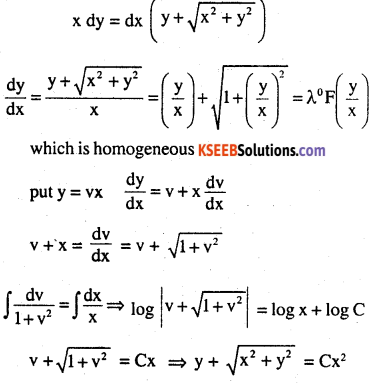Question 8.
Solve
$$\left(y+3 x^{2}\right) \frac{d x}{d y}=x$$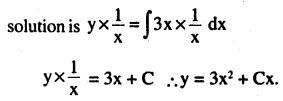Question 9.
Solve x dy + (y – x3) dx = 0 (CBSE 2011)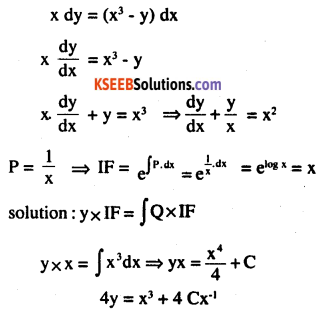Question 10.
Form the differential equation of the firmly of circles in the second quadrant and touching the co-ordinate axes. (CBSE 2012)
Centre is (-h, h), v = h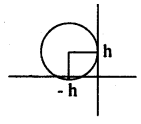equation of circle is (x + h)2 + (y – h)2 = h2
(x – h)2 + (y – h)2 = h2 dy
2(x – h) +2(y – h) $$\frac{d y}{d x}=0$$Question 11.
(1 – x)2 dy + 2 xy dx = cot x dx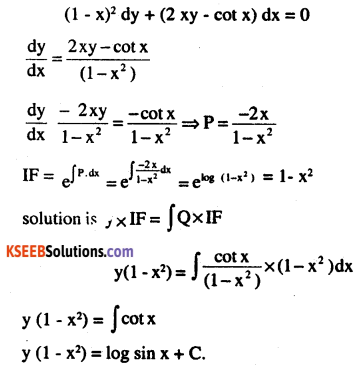$$\int x\left(x^{2}-1\right) \frac{d x}{d y}=1, y=0, \text { when } x=2$$ (CBSE 2012)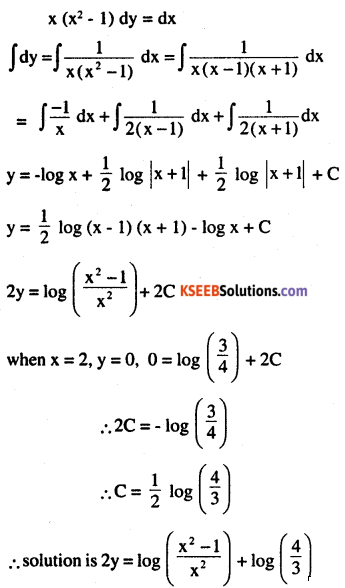$$x \cfrac{d y}{d x}-y+x \sin \left(\cfrac{y}{x}\right)=0, \text { when } x=2, y=\pi$$